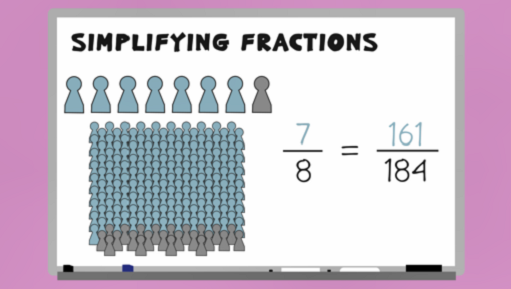Everyday maths 2

Start this free course now. Just create an account and sign in. Enrol and complete the course for a free statement of participation or digital badge if available.

Free course

# 4.2 Simplifying fractions

Watch the video below which looks at how to simplify fractions before having a go yourself in Activity 7.Skip transcript

#### Transcript

In this video, you'll look at how to simplify fractions. You'll be used to seeing the results of company surveys given as fractions: '7/8 people say they were satisfied with our customer service'. This is an example of a fraction in its simplest form. It could be that 184 people were surveyed and that 161 of them responded to say they were satisfied. Although they are equivalent fractions, you can see that the fraction '7/8 people' is a lot more user friendly than saying 161/184 people responded to say they were satisfied.

If you're asked to give an answer as a fraction in its simplest form, you firstly find a number that you can divide both parts of the fraction by, and divide it. For example, to simplify the fraction 12/18, you can divide both the top and the bottom number by 2, to give 6/9.

Keep going until you can't find a number that you can divide both parts of the fraction by. The fraction 6/9 can be divided again, this time by 3, to get 2/3. This is now the fraction in its simplest form.

Now let's simplify the fraction 40/120. Remember, find a number that you can divide both parts of the fraction by, and keep going until there is no longer a number that works. So, divide the top and bottom by 10. Divide the top and bottom by 2. Divide the top and bottom by 2. This is the fraction in its simplest form.

End transcript

Interactive feature not available in single page view (see it in standard view).

## Activity 7: Simplest form fractions

1. A local supermarket conducted a survey of 100 customers. 86 of them said they felt they got good value for money. Express this as a fraction in its simplest form.
2. 30 people entered a raffle. 6 of these people won a prize. What fraction of people did not win a prize? Give your answer as a fraction in its simplest form.

1. The initial fraction here is 86/100. This simplifies to 43/50 (divide top and bottom by 2)
2. As this question wants the number of people who did not win a prize we must first do 30 − 6 = 24 people did not win a prize.

As a fraction this becomes 24/30 which simplifies to 12/15 (divide top and bottom by 2).

This can be further simplified to 4/5 (divide top and bottom by 3).

Now that you can express a quantity as a fraction and simplify fractions, the next step is to be able to work out fractions of amounts. For example, if you see a jacket that was priced at £80 originally but is in the sale with 2/5 off, it’s useful to be able to work out how much you will be paying.

FSM_2